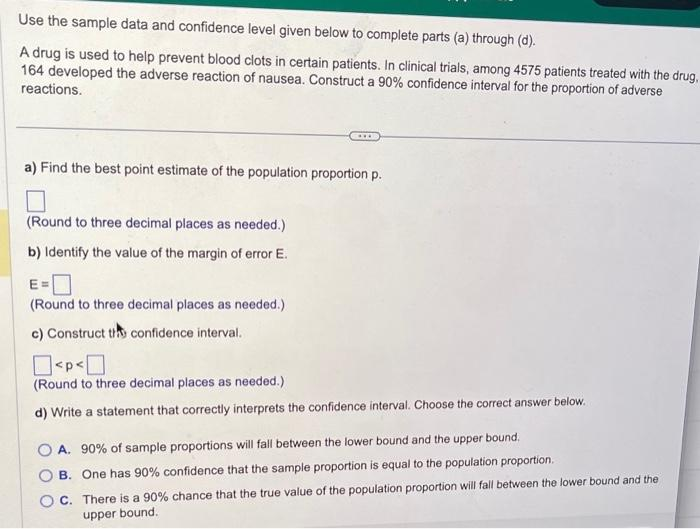# (Solved): parts a-d pleaseee Use the sample data and confidence level given below to complete parts (a) throug ...Use the sample data and confidence level given below to complete parts (a) through (d). A drug is used to help prevent blood clots in certain patients. In clinical trials, among 4575 patients treated with the drug 164 developed the adverse reaction of nausea. Construct a confidence interval for the proportion of adverse reactions. a) Find the best point estimate of the population proportion . (Round to three decimal places as needed.) b) Identify the value of the margin of error . (Round to three decimal places as needed.) c) Construct this confidence interval. (Round to three decimal places as needed.) d) Write a statement that correctly interprets the confidence interval. Choose the correct answer below. A. of sample proportions will fall between the lower bound and the upper bound. B. One has confidence that the sample proportion is equal to the population proportion. C. There is a chance that the true value of the population proportion will fall between the lower bound and the upper bound.

We have an Answer from Expert